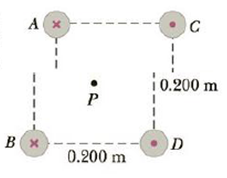Chapter 19, Problem 49P

Chapter
Section
Textbook Problem

Four long, parallel conductors carry equal currents of I = 5.00 A. Figure P19.49 is an end view of the conductors. The direction of the current is into the page at points A and D (indicated by the crosses) and out of the page at C and D (indicated by the dots). Calculate the magnitude and direction of the magnetic field at point P, located at the center of the square with edge of length 0.200 m.Figure P19.49

To determine

The magnitude and direction of magnetic field at point P.

Explanation

Given info: The four conductors are parallel and carry equal currents of magnitude I=5.00A. The direction of current at point A and B is into the page. The current at point C and D are out of the page. The edge of the square is 0.200m. The point P is at the center of the square.

The arrangement of the wires is given by the following figure.

The magnetic field due to a straight current carrying wire is given by,

B=μ0I2πr      (1)

• μ0 is the permeability of free space
• I is the current in the wire
• r is the distance of the point from the wire

The distance between the parallel conductors and the point is,

r=s2

• s is the length of the edge of the square

Hence the magnetic field is,

B=μ0I2π(s2)=μ0I2πs      (2)

The magnetic field due to the conductor at A will point to the left and down at 135°.

The magnetic field due to the conductor at B will point to the right and down at 45°.

The magnetic field due to the conductor at C will point to the right and down at 45°.

The magnetic field due to the conductor at D will point to the left and down at 135°.

The horizontal component of the magnetic field created due to the conductors at point A and C will cancel out each other.

The horizontal component of the magnetic field created due to the conductors at point B and D will cancel out each other

Still sussing out bartleby?

Check out a sample textbook solution.

See a sample solution

The Solution to Your Study Problems

Bartleby provides explanations to thousands of textbook problems written by our experts, many with advanced degrees!

Get Started

The theory that vitamin C prevents or cures colds is well supported by research. T F

Nutrition: Concepts and Controversies - Standalone book (MindTap Course List)

The metric prefix micro- means ___. (1.5)

An Introduction to Physical Science

What is an intron?

Introduction to General, Organic and Biochemistry